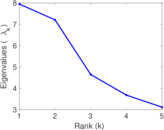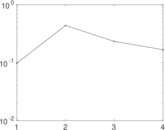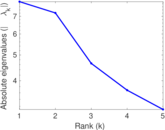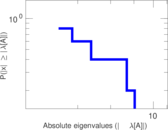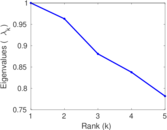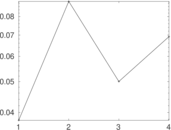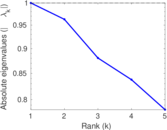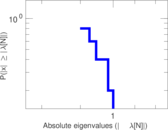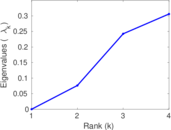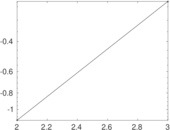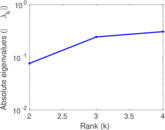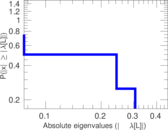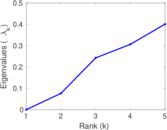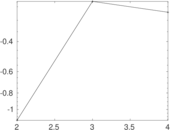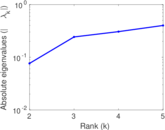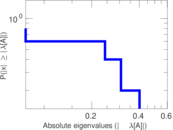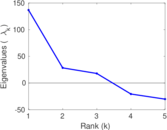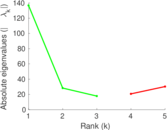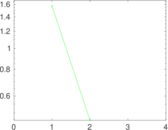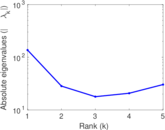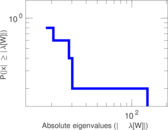# American Revolution

This bipartite network contains membership information of 136 people in 5 organisations dating back to the time before the American Revolution. The list includes well-known people such as the American activist Paul Revere. Left nodes represent persons and right nodes represent organisations. An edge between a person and an organization shows that the person was a member of the organisation.

 Code `Ar` Internal name `brunson_revolution` Name American Revolution Data source https://github.com/corybrunson/triadic AvailabilityDataset is available for download Consistency checkDataset passed all tests Category Affiliation network Node meaning Person, organization Edge meaning Membership Network formatBipartite, undirected Edge typeUnweighted, no multiple edges

## Statistics

 Size n = 141 Left size n1 = 136 Right size n2 = 5 Volume m = 160 Wedge count s = 3,433 Claw count z = 58,072 Cross count x = 756,527 Square count q = 64 4-Tour count T4 = 14,872 Maximum degree dmax = 59 Maximum left degree d1max = 4 Maximum right degree d2max = 59 Average degree d = 2.269 50 Average left degree d1 = 1.176 47 Average right degree d2 = 32.000 0 Fill p = 0.235 294 Size of LCC N = 141 Diameter δ = 6 50-Percentile effective diameter δ0.5 = 3.079 98 90-Percentile effective diameter δ0.9 = 3.825 69 Median distance δM = 4 Mean distance δm = 3.107 33 Gini coefficient G = 0.551 654 Balanced inequality ratio P = 0.296 875 Left balanced inequality ratio P1 = 0.456 250 Right balanced inequality ratio P2 = 0.300 000 Relative edge distribution entropy Her = 0.772 611 Power law exponent γ = 5.503 71 Tail power law exponent γt = 2.721 00 Tail power law exponent with p γ3 = 2.721 00 p-value p = 0.014 000 0 Left tail power law exponent with p γ3,1 = 3.391 00 Left p-value p1 = 0.196 000 Right tail power law exponent with p γ3,2 = 2.011 00 Right p-value p2 = 0.554 000 Degree assortativity ρ = −0.243 064 Degree assortativity p-value pρ = 0.001 954 75 Spectral norm α = 7.949 68 Algebraic connectivity a = 0.076 333 5 Spectral separation |λ1[A] / λ2[A]| = 1.103 10 Controllability C = 131 Relative controllability Cr = 0.929 078

## Plots

### Fruchterman–Reingold graph drawing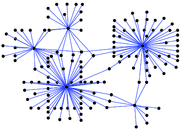### Degree distribution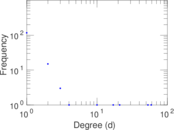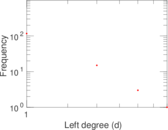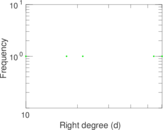### Cumulative degree distribution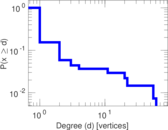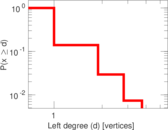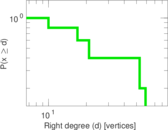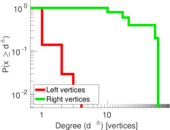### Lorenz curve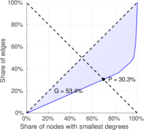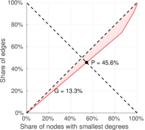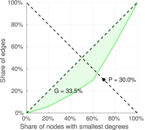### Spectral distribution of the adjacency matrix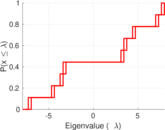### Spectral distribution of the normalized adjacency matrix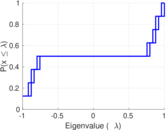### Spectral distribution of the Laplacian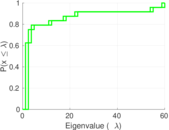### Spectral graph drawing based on the adjacency matrix### Spectral graph drawing based on the Laplacian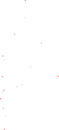### Spectral graph drawing based on the normalized adjacency matrix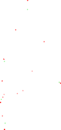### Degree assortativity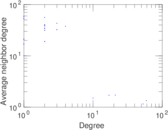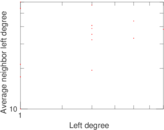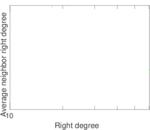### Zipf plot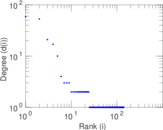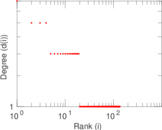### Hop distribution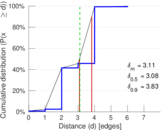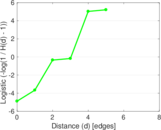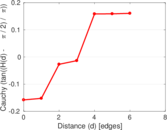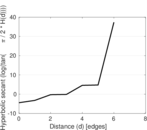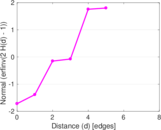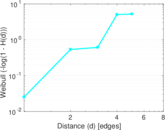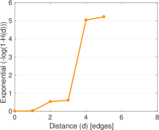### Double Laplacian graph drawing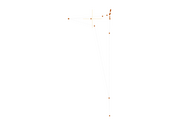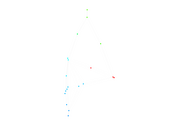### Delaunay graph drawing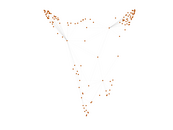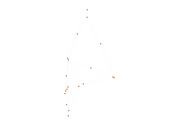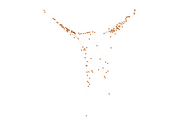### Matrix decompositions plots